Reach Us+44-7482-875032
Classifying Users of a Topic Recommendation System with a Restricted Boltzmann Machine with Nearest Neighbor Interactions | OMICS International
Journal of Applied & Computational Mathematics
All submissions of the EM system will be redirected to Online Manuscript Submission System. Authors are requested to submit articles directly to Online Manuscript Submission System of respective journal.

# Classifying Users of a Topic Recommendation System with a Restricted Boltzmann Machine with Nearest Neighbor Interactions

James Tesiero*

University of Maine, Orono, USA

*Corresponding Author:
James Tesiero
Principal Data Scientist Consultant
University of Maine, Orono, USA
Tel: (508)845-9448
E-mail: [email protected]

Received Date: January 06, 2015; Accepted Date: May 13, 2015; Published Date: May 20, 2015

Citation: Tesiero J (2015) Classifying Users of a Topic Recommendation System with a Restricted Boltzmann Machine with Nearest Neighbor Interactions. J Appl Computat Math 4:218. doi: 10.4172/2168-9679.1000218

Copyright: © 2015 Tesiero J. This is an open-access article distributed under the terms of the Creative Commons Attribution License, which permits unrestricted use, distribution, and reproduction in any medium, provided the original author and source are credited.

Visit for more related articles at Journal of Applied & Computational Mathematics

#### Abstract

In this work, we discover groups of similar users of a topic recommendation system. The data sets used are from Tipster Newsgroups, which are used in the annual TREC (Text Retrieval Conference) competition. The users are simulated, represented by tag/rating pairs. The documents in the Tipster data sets are clustered with a topic clustering algorithm that is a subset of the topic recommendation system being proposed in this paper. The users query the clusters derived from the topic clustering algorithm with tags, then rate the degree of relevance the content returned by the system has to their tag. It is shown in this work that, starting from a random sampling of the clusters by the users, and a random initial distribution of ratings per user, that a topic recommendation engine powered by a Boltzmann machine with nearest neighbor interactions results in two distinct clusters of users: those that converge quickly to a particular single topic, and those who explore a few different topics in a way that is periodic in time. This allows new users entering the system to be clustered and hence given a more relevant experience earlier in the process.

#### Keywords

Topic clustering; Cluster entropy; Information retrieval; Boltzmann machines

#### Introduction

The problem of topic recommendation applied to an evolving set of users is a subset of the general problem of user classification. Here, we focus on user acquisition of knowledge due to an evolving set of questions based on the interaction of users of various topic interests and levels of expertise. The structure of this paper is as follows. First, we describe the business problem which gives context to the problem we are solving. Next, the data sets and how they are used in the model is described. We then explain the components of the model, first the topic clustering followed by the user simulation/topic recommendation process. The findings of this research and their significance are then discussed, and the article finishes with a description of future work stemming from this research.

Brief review of the model

Recommendation systems fall into three main categories:

1) Content based recommendation systems

2) Collaborative Filtering Techniques

3) Hybrid Recommendation Systems

This model falls into the hybrid category since it contains aspects of both content (via the document clustering model) and collaborative filtering (using ratings of other users). However, it is unique in the hybrid category as it is the first system to our knowledge that combines a Restricted Boltzmann Machine with a fuzzy clustering technique to define a single new metric (the CR divergence) to classify users based on their content selection, rating of that content, and topic exploration behavior.

Business problem

The business problem that is addressed in this paper is that of increasing the quantity and quality of shared knowledge in the topic areas, and identifying sources of authority as well as building shared knowledge between related topic areas. The implementation of this concept is an intelligent database of knowledge and users that evolves over time and continuing user interaction.

In this paper, we discuss the simulation of this intelligent database, through the use of simulated users. A simulated user in our system is represented by a tag-rating pair. The tag represents a query to the database of knowledge stored in the clustered content which results from applying our supervised topic clustering algorithm to the data. We use inverse cluster entropy as a metric of similarity; from this the probability distribution of cluster membership for the tag is found.

In real-time, new users will enter the system at different rates, and existing users are likely to return. Also, different documents in the content clusters will be visited multiple times. Since each user is represented by a tag-rating pair, and a tag is a query of the clustered database, then each user effectively is represented by the probability distribution of cluster membership given by its tag and the ratings that it gives the items returned as a result of the query.

The users then, form a graph over the underlying content clusters. From the ratings of content items by the users and their nearest neighbors, and the distance on the graph, a cost function is derived which determines the next tag used to query the clusters.

The next tag can either be from the same user at a different time or another user in the same user cluster. This tag then results in more content being displayed to the user (or user in the same cluster), which is in turn rated, and results in a new set of nearest neighbors.

The users enter and exit the system in batches. Each batch represents a new time step in the simulation, with a noise parameter introduced analogous to the inverse temperature in simulated annealing applications. It is shown that at a critical value of the noise parameter, the system becomes ordered into at least two distinct user modes.

Data sets

The data sets used for this work were from the Tipster Newsgroup Collection. These data sets are used in the annual TREC competition (Text Retrieval Competition) centered on the TREC conference. There were 10 K documents selected randomly from 20 different topic areas used in this research. The topic areas were the following: atheism, computer graphics, computer operating systems, computer PC hardware, computer MacIntosh hardware, computer windows OS, miscellaneous, automobiles, motorcycles, baseball, hockey, encryption, electronics, medicine, space, Christian religion, guns, Middle East politics, general politics, and general religion. They covered the broad topic areas of religion, science, politics, computers, and sports. The documents in the Tipster dataset were classified manually by a team of human editors.

Related work

Other research related to this work are:  “A Unified Approach to Building Hybrid Recommendation Systems”, A. Guadawardana and C. Meek, which describes a method of classifying users based on a Restricted Boltzmann Machine , “Recommendation as Classification: Using Social and Content Based Information in Recommendation”, C. Basu, H. Hirsh, and W. Cohen, which uses hybrid features that contain elements of content and rating behavior to classify users.

Components of the Model

We now describe the components of the model. First, the topic clustering model is discussed. This model clusters the documents comprising the Tipster data collection. Then, the Boltzmann Machine is described, which simulates the users with tag-rating pairs that query the clusters for content, then rate that content, and share the content with their nearest neighbors as defined by the Boltzmann Machine .

Topic clustering

The first step in classifying users that query a clustered corpus to obtain, rate, and share content is to cluster the documents in the corpus. In order to do this, there must be a model of the documents that represents them in an N dimensional space. The model that we use to do this is used often in information retrieval, the tf-idf model (term frequency-inverse document frequency). However, we define the term frequency and the inverse document frequency in a different manner than is often encountered in the information retrieval literature. We also define the cluster term frequency and use it to filter terms that have little or no discriminative power.

Term frequency: The standard definition of the term frequency is the relative number of times a term in the corpus of documents appears in a particular document.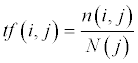(1)

(Where tf(i,j) is the term frequency of the ith term in the jth document, n(i,j) is the number of times that the ith term appears in the jth document, and N(j) is the total number of terms in the jth document)

There are two significant problems with this definition, in particular with short documents. First, for terms that occur at least once in short documents, the formula overestimates the probability because there are fewer discrete choices.

For example, in a twelve term document, if a term appears once, according to the above formula its term frequency is 0.083, which is in the 90th percentile at least in most corpuses. The second problem associated with the simple definition above is that for terms those do not appear at all in short documents, they potentially may have appeared if the document was longer.

We can exploit the fact that the documents have been pre-classified by treating documents in the clusters as if they were random selections of terms in their cluster, where the document length is also a random variable. The term frequency can be treated as a state whose value depends upon the selection (document) from the reservoir (cluster).

Our definition of the term frequency, which normalizes the frequency to document length, is as follows: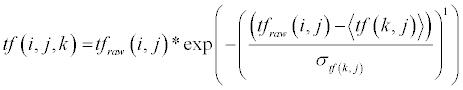(2)

where tfraw(i,j) is the term frequency as it is usually defined in (1) above, k denotes the cluster that the Ith document that contains the jth term is in, <tf(k,j)> is the mean term frequency for the jth term in the kth cluster over the documents in that cluster other than the ith document. σtf (k,j) denotes the standard deviation of the term frequency for the jth term in the kth cluster over the documents in that cluster other than the ith document.

Weighted inverse document frequency: The inverse document frequency as typically defined in the information retrieval literature is: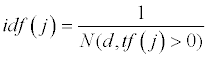(3)

(where idf(j) is the inverse document frequency of the jth term in the corpus, and N(d,tf(j)>0) is the number of documents in the corpus where the raw term frequency is greater than zero (or, all of the documents where that term occurs at least once))

However, the occurrence of a term just once, especially in a longer document, can occur by chance. This artificially lowers the idf and makes a term appear less relevant than it actually is. We define an inverse document frequency metric that is robust to noise fluctuations in term occurrence.

We do this by again exploiting the fact that we have a supervised classification of the documents. This allows us to define a cluster term frequency. The cluster term frequency is similar in form to the raw term frequency, except that we count the relative frequency of terms in a particular cluster.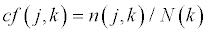(4)

(where cf(j,k) is the cluster frequency of the jth term in the kth cluster, n(j,k) is the number of occurrences of the jth term in the kth cluster, and N(k) is the number of terms in the kth cluster)

With the above definition of the cluster term frequency, we can build a definition of the idf that conceptually treats documents in the same cluster as equivalent and those outside as different. The cluster term entropy can be defined as the following, when the cluster frequency is interpreted as a probability: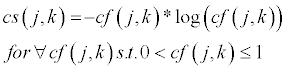(5)

The value at cf(j,k) = 0 is excluded since the logarithm diverges to negative infinity there. We define a smoothed inverse cluster entropy to avoid divergence when cf(j,k) = 1 as the following:

ics( j,k ) = exp(−cs( j,k ))                     (6)

This equation yields a value for ics(j,k) near 1 when the entropy is low (when the term is highly discriminating between the clusters), and near 0 where the entropy is high (a uniform distribution over the clusters), and without diverging anywhere. This metric is used to weight the traditional idf (equation 3) to produce a weighted idf:

widf ( j,k ) = ics( j,k )*idf ( j)                   (7)

which is then multiplied by the robust term frequency (equation 2) to obtain the relevance score:

rel (i, j,k ) = tf (i, j,k )*widf ( j,k )              (8)

This equation yields the relevance of the jth term to the ith document in the kth cluster. The relevance is then summed over the terms in a document and used in a logistic equation to calculate the probability of the ith document in the kth cluster.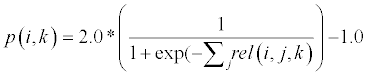(9)

Since rel(i,j,k) is constrained to lie between zero and one, we use the constants in the equation (9) above to allow p(i,k), which is the probability of the ith document in the kth cluster, to lie between 0 and 1 inclusive. The probability distribution of each document over the clusters is then put into a graph, which serves as the content database which simulated users query with tags.

Simulating users

Now that we have demonstrated how documents get classified, we turn our focus to the user querying process, and the rating and sharing of content amongst users.

Ratings map and distance function: Each user is simulated as a tag-rating pair. The user queries the clusters with a tag and is returned a set of documents to rate (in the most general case, the documents can be other tags). The ratings are done with the original Netflix system (scale from 1-5), which is then mapped to a binary variable (like/dislike). Netflix ratings from 1-3 map to -1 (dislike) and ratings 4-5 map to 1 (like). The documents that are returned for rating are based on the highest document probability conditioned on the cluster frequency of the user tag.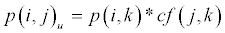(10)

(where p(i,k) is given by equation (9) and cf(j,k) is given by equation (4), and p(i,j)u is the probability of the ith document given the jth term associated with the tag of the uth user)

For each user making a query to the clusters, we return the top three documents. These correspond to the documents having the top three values of p(i,j)u in equation (10) above. The users are related to each other by the probability distribution across the clusters created by their tag. For example, if there are C clusters, then users A and B lie on the following points of the C dimensional graph at time t:

User A: {cf(tag(A(t)),1),cf(tag(A(t)),2),…,cf(tag(A(t)),C)}

User B: {cf(tag(B(t)),1),cf(tag(B(t)),2),…,cf(tag(B(t)),C)}

Using the city block distance metric, we can construct a C dimensional graph such that the nearest neighbors of any user on the graph at any time can be calculated.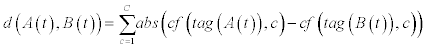(11)

Using the above distance function, we can construct a graph for the users of the system at any point in time. The nearest neighbors of a user have an influence on the user that is inversely related to the above distance in the following way: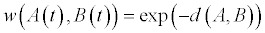(12)

Where w(A(t),B(t)) is the mutual influence that users A and B have on each other. The symmetry of the weight function is directly related to the symmetry of the distance function (11). This weight function has two nice properties: when A and B are the same user at the same time, the value is 1 (which is the maximum value it can have), and since the weight function goes to zero asymptotically, large numbers of nearest neighbors can still have some small influence.

Cost and partition functions: In this section, we develop the concepts of the cost and partition functions, and use them to define the metric which will be used to classify the users, the CR divergence (content-rating divergence).

The pairwise cost between users A and B above (or any two users of the system at any time) is defined as: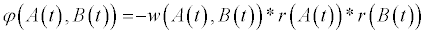(13)

where φ(A(t),B(t)) is the cost of putting users A and B in the same neighborhood, w(A(t),B(t)) is the weight, or influence of user B on A as defined in (12), and r(A(t)),r(B(t)) are the ratings given to the content seen by users A and B at time t. Note that the larger the weight, the greater the effect on the cost function for any two users at any time. Since the goal is to minimize the cost, with the presence of the minus sign in (13) the larger the weight, the lower the pairwise cost becomes. Also, users that give the same rating lower the cost function, while those giving opposite ratings raise it.

The cost function as defined above is between a single nearest neighbor B with A; if we sum this over all nearest neighbors, we obtain the single user cost φ (A(t)) defined as the following: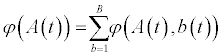(14)

Where b=1,…,B are the nearest neighbors of A

The single user cost function (14) is then used to derive a single user partition function, with a Boltzmann probability distribution.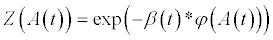(15)

Where β(t) is an inverse noise parameter and φ(A(t)) is as defined in

Substituting equations (13) and (14) in (15) expresses the single user partition function in terms of the influence and ratings of its nearest neighbors at that time: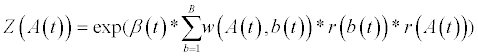(16)

The sum over the product of the weights and the ratings of the nearest neighbor’s yields a metric we call the content-rating divergence (or, CR divergence).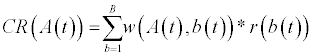(17)

The CR divergence can be conceptualized as a “mean field” on the user A at time t. If the neighboring users that like content similar to that viewed by user A at time t have more influence as measured by the weight function at that time than neighboring users that dislike content similar to that viewed by user A at time t, then the overall CR divergence is positive, for example.

Substituting the CR divergence (17) into the single user partition function (16), we have: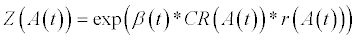(18)

Since the users are acting independently of each other, the partition function Z(G(t)) over the graph at time t can be written as a product of single user partition functions.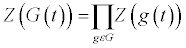(19)

User classification: In this section, we use the cost and partition function derived above to show how users can be classified with the correlation of their CR divergences.

The free energy function is related to the partition function by the equation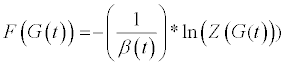(20)

The partition function Z(g(t)) for a single user at a particular time has two possible states, corresponding to the two possible ways the user can rate the content item being viewed at that time. Therefore, the explicit form of Z(G(t)) is: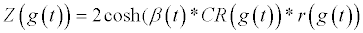(21)

Substituting this explicit form of the single user partition function into equation (20) and differentiating with respect to r yields: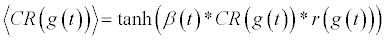(22)

Since this quantity can be calculated anywhere on the graph, the correlation between any two points on the graph can be measured. The points on the graph represent a single user at a particular time, so the two user partition function can be written as: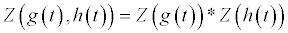(23)

The mixed partial derivative of this partial derivative with respect to g(t),h(t) then yields the correlation between the users corresponding to those points: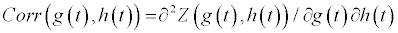(24)

Finding the zeros of this equation between all pairwise comparisons on the graph yields boundaries separating different user classes. In the beginning of the simulated process, the “inverse temperature” parameter β is close to 0 (analogous to high temperature in physical systems). This yields a two point correlation function that is zero almost everywhere. As the simulated annealing process evolves and β increases, two classes of users form from the boundary that forms on the graph, creating two distinct regions. The boundary separates users that focus in a single topic area from those that periodically visit multiple, but related topic areas. Data to support these conclusions are provided in the Findings section below.

#### Findings and Conclusions

Using the Tipster data set alluded to previously, and the model developed by the author, two distinct user classes have been discovered in a simulated set of 1000 users. These classes emerge at a critical beta value that occurs during the simulated annealing process. One of the classes consists of users who focus on a particular topic, while the other consists of users that periodically hop between different topics. The users in both classes started from an initial state in which they received random content, and were producing random ratings (Table 1).

 3 3 3 3 3 3 3 3 3 3 3 3 3 3 3 3 3 3 3 3 3 3 3 3 3 3 3 3 3 3 6 6 6 6 6 6 6 6 6 6 6 6 6 6 6 6 6 6 6 6 6 6 6 6 6 6 6 6 6 6 12 11 14 12 11 14 12 11 14 12 14 12 11 14 12 11 14 12 11 14 20 17 20 17 20 17 20 17 20 17 11 14 12 11 14 12 11 14 12 11

Table 1: Clusters visited by members of the user groups (rows are User Groups).

The data below are from the final 100 users in the data set, after the critical value of beta was reached and the graph was close to equilibrium. Of these users, 60 were single topic focused users, split evenly between the computer operating systems content cluster and computer windows operating systems content cluster. The remaining 40 users consisted of two subgroups. There were 30 users who hopped periodically between hockey, encryption, and medicine, and 10 users who hopped periodically between guns and religion. But both subgroups demonstrated a periodic time dependence, hence they were classified as the same user group although the content that they sampled was different. Because of the simple types of time dependence (static and periodic), it is practical and relatively simple to classify new users in the system, based on their CR divergence, which is shown in the graph below (Figure 1).

The cells in the chart above refer to the cluster visited by a user at a particular time. The numbers are in the order referenced in the Data Sets section above. Note the periodic pattern in the last 4 rows.

The user groups referred to in the CR divergence graph correspond to the rows from top to bottom in the equilibrium visitor trajectories chart. Note that there is a clear distinction between user groups 1-6 that have a CR divergence different than zero, and positive, while groups 7-10 have a CR divergence statistically equivalent to zero. This demonstrates the two phases of user classification alluded to previously.

A comparison of the performance of this model to related models mentioned in the Related Work section is difficult due to the use of simulated users in this work, even if the same content collection (the Tipster data set collection) was used.

#### Future Works

The scope of future work is twofold at this point. First, we will further study how the algorithm and grouping of users evolves with greater scale of users and content, and with increased content and user diversity. The second focus will be to study what happens more closely at the critical value of beta, where the transition into clusters actually takes place, to better understand that process.

#### References

Select your language of interest to view the total content in your interested language

### Article Usage

• Total views: 12085
• [From(publication date):
June-2015 - Aug 21, 2019]
• Breakdown by view type
• HTML page views : 8309
• PDF downloads : 3776

## Post your commentCan't read the image? click here to refresh
###### Peer Reviewed Journals

Make the best use of Scientific Research and information from our 700 + peer reviewed, Open Access Journals

International Conferences 2019-20

Meet Inspiring Speakers and Experts at our 3000+ Global Annual Meetings

Top